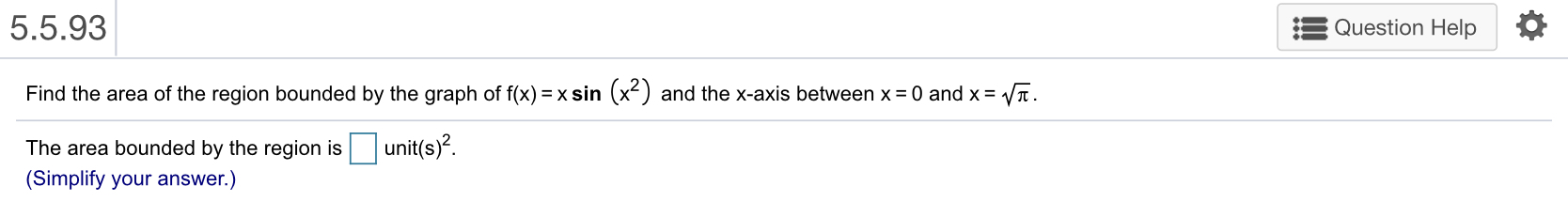# 5.5.93Question HelpFind the area of the region bounded by the graph of fx)- xsin (x2) and the x-axis between x 0 and xV.The area bounded by the region isunit(s)-.(Simplify your answer.)

Question
1 viewshelp_outlineImage Transcriptionclose5.5.93 Question Help Find the area of the region bounded by the graph of fx)- xsin (x2) and the x-axis between x 0 and xV. The area bounded by the region isunit(s)-. (Simplify your answer.) fullscreen
check_circle

Step 1

The given function is f(x) = x sin(x2).

Step 2

Change the limits as follows.

Step 3

Obtain the area a...

### Want to see the full answer?

See Solution

#### Want to see this answer and more?

Solutions are written by subject experts who are available 24/7. Questions are typically answered within 1 hour.*

See Solution
*Response times may vary by subject and question.
Tagged in

### Integration